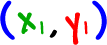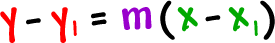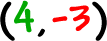The next thing is to remember how to find the equation of a line given a point and a slope.

 If we have a point,and a slope, m, here's our formula:You'll be using this a lot, so let's run through a couple of examples...

 Find the equation of the line that passes through the pointwith a slope of -2: